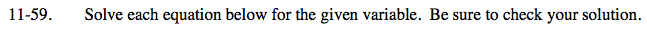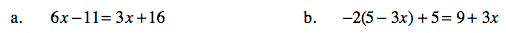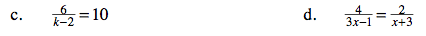### Home > CAAC > Chapter 11 > Lesson 11.1.5 > Problem11-59

11-59.6x = 3x + 27

Subtract 3x from both sides.

3x = 27

Solve for x.

x = 9

Distribute the −2.

Follow the steps in part a.Multiply both sides by a common factor of the denominators to remove the fractions.

6 = 10( k − 2 )

Follow the steps in part b.

Follow the steps in part c.

x = 7